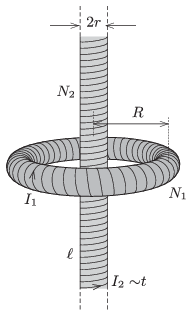Mathematical and Physical Journal
for High Schools
Issued by the MATFUND Foundation
 Already signed up? New to KöMaL?

#Problem P. 4800. (January 2016)

P. 4800. The electric current in a toroid of mean radius $\displaystyle R$ is $\displaystyle I_1$, and its number of turns is $\displaystyle N_1$. A solenoid of radius $\displaystyle r$ and of length $\displaystyle \ell$ ($\displaystyle \ell\gg R$), is placed along the symmetry axis of the toroid. The number of turns of the solenoid is $\displaystyle N_2$. In this coil the current is increased uniformly during a time of $\displaystyle t_0$, until the magnetic field in the toroid

$\displaystyle a)$ becomes zero;

$\displaystyle b)$ becomes the opposite of the original magnetic field in the toroid.

Determine the electric field strength in the toroid along its mean radius in both cases.Data: $\displaystyle R=20$ cm, $\displaystyle N_1=300$, $\displaystyle r=4$ cm, $\displaystyle \ell=2$ m, $\displaystyle I_1=0.5$ A, $\displaystyle N_2=4000$, $\displaystyle t_0=2$ s.

(5 pont)

Deadline expired on February 10, 2016.

### Statistics:

 19 students sent a solution. 5 points: Asztalos Bogdán, Balogh Menyhért, Bekes Nándor, Blum Balázs, Büki Máté, Forrai Botond, Iván Balázs, Kasza Bence, Kormányos Hanna Rebeka, Kovács Péter Tamás, Németh 777 Róbert, Sal Kristóf, Szentivánszki Soma , Tomcsányi Gergely, Zöllner András. 4 points: Csorba Benjámin, Fekete Balázs Attila, Körmöczi Dávid, Németh Flóra Boróka.

Problems in Physics of KöMaL, January 2016